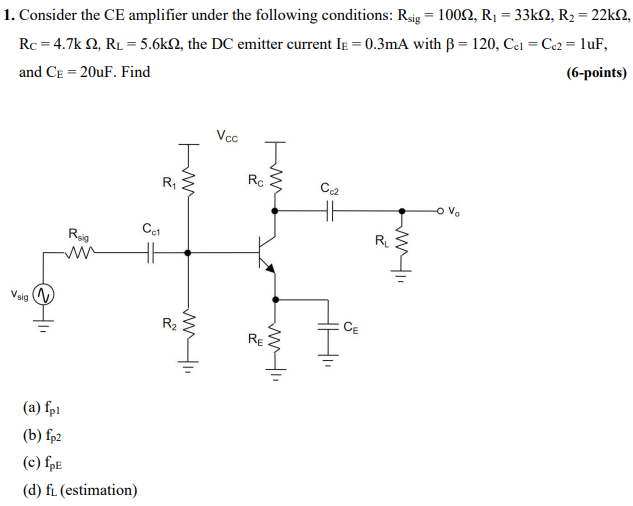# Question = 1. Consider the CE amplifier under the following conditions: Rsig = 10012, R2 = 33KS2, R2 = 22KN, Rc = 4.7k 2, RL = 5.6k12, the DC emitter current le = 0.3mA with B = 120, Cei = Cc2 = 1uF, and CE = 20uF. Find (6-points) Vcc T w اله R اللي Ro C2 OV Rsig Cct RL 4 Vsig R2 CE W1 RE wth (a) fp1 (6) fp2 (c) fpE (d) fl (estimation)V2HEW4 The Asker · Electrical EngineeringTranscribed Image Text: = 1. Consider the CE amplifier under the following conditions: Rsig = 10012, R2 = 33KS2, R2 = 22KN, Rc = 4.7k 2, RL = 5.6k12, the DC emitter current le = 0.3mA with B = 120, Cei = Cc2 = 1uF, and CE = 20uF. Find (6-points) Vcc T w اله R اللي Ro C2 OV Rsig Cct RL 4 Vsig R2 CE W1 RE wth (a) fp1 (6) fp2 (c) fpE (d) fl (estimation)
More
Transcribed Image Text: = 1. Consider the CE amplifier under the following conditions: Rsig = 10012, R2 = 33KS2, R2 = 22KN, Rc = 4.7k 2, RL = 5.6k12, the DC emitter current le = 0.3mA with B = 120, Cei = Cc2 = 1uF, and CE = 20uF. Find (6-points) Vcc T w اله R اللي Ro C2 OV Rsig Cct RL 4 Vsig R2 CE W1 RE wth (a) fp1 (6) fp2 (c) fpE (d) fl (estimation)